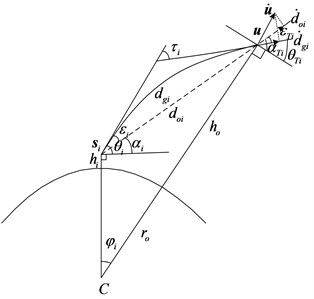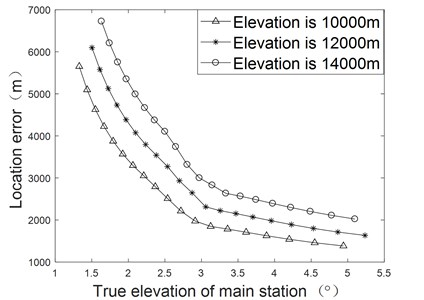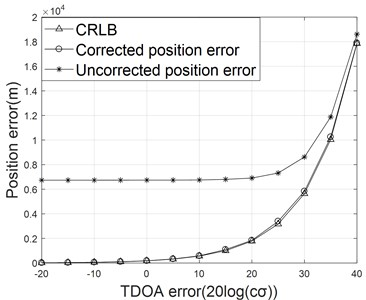Vibroengineering PROCEDIA

Published: 12 December 2022

# TDOA positioning in the presence of atmospheric refraction and observer uncertainty influence analysis and correction

Yanlin Wu1
Shuqiang Zhang2
Wei Zhu3
Zongzhe Nie4
1, 2, 3, 4College of Information and Communication, National University of Defense Technology, Wuhan, 430010, China
Corresponding Author:
Shuqiang Zhang
Views 8

#### Abstract

Due to the inhomogeneity of the atmosphere, radio waves are subjected to refraction during the propagation process, which reduces its propagation speed and bends the propagation path. If the influence is not considered, a large error will be caused by using time difference of arrival (TDOA). The influence of atmospheric refraction on the TDOA localization under the known elevation constraint is analyzed, a subsection iterative method is proposed to correct the localization error caused by atmospheric refraction, the Cramer-Rao lower bound (CRLB) for location estimation under atmospheric refraction is deduced. The effectiveness of the proposed method is validated through simulation results and analysis.

## 1. Introduction

In the actual battlefield environment, most of the moving radiation sources are flying at equal altitude and parallel to the Earth's surface . By resorting to this constraint, the position of moving sources can be estimated accurately and simultaneously by using TDOA measurements from only three stations. Due to the reduced propagation speed of radio signal in the atmosphere and the influence of uneven atmospheric refraction, atmospheric refraction error will appear in the measured time difference, resulting in position error.

In single-station active radar applications, distance, azimuth and elevation angles are utilized, and the atmospheric refraction errors can be calculated directly according to the explicit atmospheric refraction model. Due to the different target positioning systems, the atmospheric refraction error correction method of single station active radar is not suitable for multi station TDOA positioning system. In order to improve the convergence speed and accuracy, a piecewise refraction model is innovatively proposed. Based on the stability of the error difference of atmospheric refraction TDOA, an iterative correction algorithm of successive approximation is proposed to correct the atmospheric refraction error of TDOA location, and analyze the impact of atmospheric refraction on the location accuracy under different random noises. To a certain extent, it can meet the requirements of modern military high-precision positioning.

## 2. Problem formulation

As shown in Fig. 1, the moving source radiates signals at the unknown position of $\mathbf{u}=\left(x,y,z{\right)}^{T}$, with its altitude given by ${h}_{o}$. A total of three observers are introduced to collect the radio signals emitted from the source. The position of the ith static observer is ${\mathbf{s}}_{i}=\left({x}_{i},{y}_{i},{z}_{i}{\right)}^{T}$, 1, 2, 3, with the altitude of the ith observer given by ${h}_{i}$. The apparent elevational angle, which is essentially the angel between the tangent direction and horizontal direction of the signal propagation path at the receiving station $i$, is denoted by ${\theta }_{i}$, while the unknown true elevational angle ${\alpha }_{i}$. The apparent elevational angle at the radiation source is ${\theta }_{Ti}$, while the true elevational angle is ${\alpha }_{Ti}$, with the elevational angle error given by ${\epsilon }_{Ti}$. The center of the earth is denoted by $C$, the radius of the earth by ${r}_{o}$, the linear propagation distance from the radiation source to the receiving station by ${d}_{oi}$, and the actual propagation path (curved distance) by ${d}_{gi}$. ${n}_{T}$ is the refractive index at the radiation source, $c$ is the speed of light, ${f}_{o}$ is the carrier frequency of the radiation source, and the wavelength is $\lambda =c/{f}_{o}$.

As the atmospheric variations in the vertical direction are of several orders larger in magnitude than those in the horizontal direction, the atmosphere refraction could thus be considered as the spherical stratification, the refractive index can be simplified to a quantity that varies only with elevation $h$, namely $n=n\left(h\right)$.The atmospheric refractive index $n$ is close to 1, the ground value is about 1.00026-1.00046. For instance, the value of $n$ at the elevation of 9 km is about 1.000105 . Another physical quantity of refraction index is introduced, namely:

1
$N\left(h\right)=\left(n\left(h\right)-1\right)×1{0}^{6},$

where $N\left(h\right)$ is the refraction index at altitude $h$.

The model error of piecewise model is proved to be relatively small, thus this model is adopted in this paper, which is given as:

2

where ${h}_{s}$ is the altitude of the ground, ${N}_{o}$ is the refraction index of the ground, and $\mathrm{\Delta }{N}_{1}$ is the gradient of the refraction index when . ${N}_{1}$ is the refraction index at the altitude km, ${c}_{\alpha 1}$ is the exponential attenuation rate from 1 km to 9 km over the ground, and ${N}_{9}$ is the refraction index at the altitude of 9 km. ${c}_{\alpha 9}$ is the exponential decay rate of altitude between 9 km-60 km. The curved propagation time from the source to station $i$ is ${t}_{gi}=\frac{1}{c}{\int }_{{h}_{i}}^{{h}_{o}}g\left(h\right)dh$, the apparent distance from the radiation source to the receiving station $i$ is:

3
${d}_{ei}={\int }_{{h}_{i}}^{{h}_{o}}g\left(h\right)dh,$

where, $g\left(h\right)=\frac{n\left(h{\right)}^{2}\left({r}_{o}+h\right)}{\sqrt{n\left(h{\right)}^{2}\left({r}_{o}+h{\right)}^{2}-{{n}_{i}}^{2}\left({r}_{o}+{h}_{i}{\right)}^{2}\mathrm{c}\mathrm{o}{\mathrm{s}}^{2}{\theta }_{i}}}$, ${n}_{i}$ is the index of refraction at altitude ${h}_{i}$. ${\theta }_{i}$ is the apparent elevational angle of the receiving station $i$, the Euclidean distance from the radiation source to the receiving station $i$ is:

4
${d}_{oi}=‖\mathbf{u}-{\mathbf{s}}_{i}‖,$

with the propagation time given by:

5
${t}_{oi}=\frac{{d}_{oi}}{c}.$

The true elevational angle of the radiation source in the station-center coordinate system at the receiving station $i$ is :

6
${\alpha }_{i}=-\mathrm{a}\mathrm{r}\mathrm{c}\mathrm{s}\mathrm{i}\mathrm{n}\left[\frac{{d}_{oi}^{2}+\left({r}_{o}+{h}_{i}{\right)}^{2}-\left({r}_{o}+{h}_{o}{\right)}^{2}}{2{d}_{oi}\left({r}_{o}+{h}_{i}\right)}\right].$

Take ${\alpha }_{i}$ as the initial value of ${\theta }_{i}^{\left(k\right)}$ in the following iteration process. Geocentric angle is:

7
${\phi }_{i}={\int }_{{h}_{i}}^{{h}_{o}}f\left(h\right)dh,$

where, $f\left(h\right)=\frac{{n}_{i}\left({r}_{o}+{h}_{i}\right)\mathrm{c}\mathrm{o}\mathrm{s}{\theta }_{i}^{\left(k\right)}}{\left({r}_{o}+h\right)\sqrt{n\left(h{\right)}^{2}\left({r}_{o}+h{\right)}^{2}-{{n}_{i}}^{2}\left({r}_{o}+{h}_{i}{\right)}^{2}\mathrm{c}\mathrm{o}{\mathrm{s}}^{2}{\theta }_{i}^{\left(k\right)}}}$. The true elevational angle at the receiving station $i$ can be obtained:

8
${\stackrel{-}{\alpha }}_{i}^{\left(k\right)}=\mathrm{a}\mathrm{r}\mathrm{c}\mathrm{t}\mathrm{a}\mathrm{n}\left[\frac{\left({r}_{o}+{h}_{o}\right)\mathrm{c}\mathrm{o}\mathrm{s}{\phi }_{i}^{\left(k\right)}-\left({r}_{o}+{h}_{i}\right)}{\left({r}_{o}+{h}_{o}\right)\mathrm{s}\mathrm{i}\mathrm{n}{\phi }_{i}^{\left(k\right)}}\right].$

The elevation angle error is ${\epsilon }_{i}^{\left(k\right)}={\theta }_{i}^{\left(k\right)}-{\stackrel{-}{\alpha }}_{i}^{\left(k\right)}$. Thus, the apparent elevation angle can be approximated as ${\theta }_{i}^{\left(k+1\right)}={\alpha }_{i}+{\epsilon }_{i}^{\left(k\right)}$, combining Eq. (7), and (8), one could obtain ${\theta }_{i}^{\left(k+2\right)}$ from ${\theta }_{i}^{\left(k+1\right)}$. Repeat the above iteration process until the required accuracy is satisfied or the update of parameter is within the given tolerance. Then we have ${\theta }_{i}={\theta }_{i}^{\left(m\right)}$. Note that when atmospheric refraction is taken into account, the TDOA curve propagation is ${t}_{gi1}={t}_{gi}-{t}_{g1}$, when atmospheric refraction is not considered, the TDOA of linear propagation is ${t}_{oi1}={t}_{oi}-{t}_{o1}$, the model error on TDOA is thus given by $\mathrm{\Delta }{t}_{i1}={t}_{gi1}-{t}_{oi1}$, ().

## 3. Influence of atmospheric refraction

When atmospheric refraction is not considered and is considered, the formulas of positioning measurement are:

$\left\{\begin{array}{l}{d}_{oi}-{d}_{o1}=c\cdot {t}_{oi1},\\ {\mathbf{u}}^{T}\mathbf{u}=\left({h}_{o}+{r}_{o}{\right)}^{2},\end{array}\right\$
$\left\{\begin{array}{l}{d}_{ei}-{d}_{e1}=c\cdot {t}_{gi1},\\ {\mathbf{u}}^{T}\mathbf{u}=\left({h}_{o}+{r}_{o}{\right)}^{2}.\end{array}\right\$

The formulas used in actual positioning measurement is:

9
$\left\{\begin{array}{l}{d}_{oi}-{d}_{o1}=c\cdot {t}_{gi1},\\ {\mathbf{u}}^{T}\mathbf{u}=\left({h}_{o}+{r}_{o}{\right)}^{2}.\end{array}\right\$

In actual positioning, the TDOA is measured under atmospheric refraction conditions, while the TDOA formula is used the linear propagation formulas, so the estimation error of position will certainly be caused. It is assumed that the position of the receiving stations are:

The positioning measurement errors of radiation source with elevation of 10 km, 12 km and 14 km are analyzed. The direction of motion is 30° from north to west in the local coordinate system. The simulation results are shown in Fig. 2. In the case of the same elevation, the smaller the elevation angle is, the larger the positioning measurement errors are, the higher the elevation, the larger the positioning measurement error.

Fig. 1Sketch of atmospheric refractionFig. 2Influence of atmospheric refraction on position error## 4. Position error correction

${\mathbf{d}}_{g}={\left[{d}_{g21},{d}_{g31}\right]}^{T}$ is the apparent distance difference, ${\mathbf{d}}_{g}^{o}={\left[{d}_{g21}^{o},{d}_{g31}^{o}\right]}^{T}$ is the true apparent distance difference, Then the measurement models of TDOA can be expressed as ${\mathbf{d}}_{g}=c{\mathbf{t}}_{g}={\mathbf{d}}_{g}^{o}+\mathbf{n}$, where, $\mathbf{n}={\left[{n}_{21},{n}_{31}\right]}^{T}$is the corresponding measurement noise, ${\mathbf{t}}_{g}={\left[{t}_{g21},{t}_{g31}\right]}^{T}$ is the curve arrival time difference. The noise variable is distributed independently and the mean value is zero. Its covariance matric is $\mathrm{E}\left[{\mathbf{n}}^{T}\mathbf{n}\right]={\mathbf{Q}}_{t}$, $\mathrm{E}$ is the expectation.

When there is an elevation constraint condition, the source position must be on the elevation constraint surface. In the three-station TDOA position system, there are only two TDOA formulas. There must be one point in the iteration on the elevation constraint surface to stabilize the difference of TDOA error. Therefore, the iterative correction method in the three-station TDOA position system will converge to the optimal point .

The specific process of iterative correction algorithm is as follows,

Step 1: According to the measured value of TDOA ${{\mathbf{t}}_{g}}^{\left(j\right)}$ ($j$ is the iteration number, and ${{\mathbf{t}}_{g}}^{\left(1\right)}$ is the initial measured value of TDOA), the position ${\mathbf{u}}^{\left(j\right)}$ of the radiation source is calculated by using the positioning Eq. (9);

Step 2: According to the formulas of the Section 2, the TDOA ${{\mathbf{t}}_{g}}^{\left(j\right)}$ of curve propagation and the TDOA ${{\mathbf{t}}_{o}}^{\left(j\right)}$ of straight line propagation at position ${\mathbit{u}}^{\left(j\right)}$.

Step 3: Calculating the TDOA error at position ${\mathbf{u}}^{\left(j\right)}$, get $\mathrm{\Delta }{\mathbf{t}}^{\left(j\right)}={{\mathbf{t}}_{g}}^{\left(j\right)}-{{\mathbf{t}}_{o}}^{\left(j\right)}$.

Step 4: Analyze the calculation results and decide whether to continue iteration the next step.

(1) if $j=1$, the new TDOA is calculated as ${{\mathbf{t}}_{g}}^{\left(j+1\right)}={{\mathbf{t}}_{g}}^{\left(j\right)}-\mathrm{\Delta }{\mathbf{t}}^{\left(j\right)}$, and return Step1 to continue the calculation.

(2) if $j>1$, calculating the difference between the current TDOA error and the previous TDOA error, get $\mathrm{\Delta }{{\mathbf{t}}_{c}}^{\left(j-1\right)}=\mathrm{\Delta }{\mathbf{t}}^{\left(j\right)}-\mathrm{\Delta }{\mathbf{t}}^{\left(j-1\right)}$. Then the decision is made: if $\mathrm{\Delta }{{\mathbf{t}}_{c}}^{\left(j-1\right)}<\mathrm{\Delta }{\mathbf{t}}_{TH}$ ($\mathrm{\Delta }{\mathbf{t}}_{TH}$ is the set threshold), the iteration is stopped and the final positioning result $\mathbf{u}$ is output. Otherwise, the new TDOA is calculated as ${{\mathbf{t}}_{g}}^{\left(j+1\right)}={{\mathbf{t}}_{g}}^{\left(j\right)}-\mathrm{\Delta }{\mathbf{t}}^{\left(j\right)}$, and go back to Step1 to continue the iteration.

## 5. CRLB of position estimation

The CRLB has extended the bound to incorporate constraints . Differentiation of Eq. (5), ${\mathbf{H}}_{1}d\mathbf{u}=cd\mathbf{T}$, where:

Taking the position $\mathbf{u}$ derivative of Eqs. (3) and (7). Because the position-dependent parameters are only the integral upper limit ${h}_{o}$ and the apparent elevation angle ${\theta }_{i}$, we can get:

10
$\frac{\partial {d}_{ei}}{\partial \mathbf{u}}=\frac{{n}_{T}^{2}\left({r}_{o}+{h}_{o}\right)}{\sqrt{{n}_{T}^{2}\left({r}_{o}+{h}_{o}{\right)}^{2}-{n}_{i}^{2}\left({r}_{o}+{h}_{i}{\right)}^{2}\mathrm{c}\mathrm{o}{\mathrm{s}}^{2}{\theta }_{i}}}\cdot \frac{\partial {h}_{o}}{\partial \mathbf{u}}$
11
$\frac{\partial {\phi }_{i}}{\partial \mathbf{u}}=\frac{{n}_{i}\left({r}_{o}+{h}_{i}\right)\mathrm{c}\mathrm{o}\mathrm{s}{\theta }_{i}}{\left({r}_{o}+{h}_{o}\right)\sqrt{{n}_{T}^{2}\left({r}_{o}+{h}_{o}{\right)}^{2}-{n}_{i}^{2}\left({r}_{o}+{h}_{i}{\right)}^{2}\mathrm{c}\mathrm{o}{\mathrm{s}}^{2}{\theta }_{i}}}\cdot \frac{\partial {h}_{o}}{\partial \mathbf{u}}$

Multiplying the two ends of Eq. (11) by ${n}_{i}\left({r}_{o}+{h}_{i}\right)\mathrm{c}\mathrm{o}\mathrm{s}{\theta }_{i}$, subtract from Eq. (10), get:

12

From cosine and sine theorem, we have ${d}_{oi}^{2}=\left({r}_{o}+{h}_{o}{\right)}^{2}+\left({r}_{o}+{h}_{i}{\right)}^{2}-2\left({r}_{o}+{h}_{o}\right)\left({r}_{o}+{h}_{i}\right)\mathrm{c}\mathrm{o}\mathrm{s}{\phi }_{i}$ and $\left({r}_{o}+{h}_{o}\right)\mathrm{c}\mathrm{o}\mathrm{s}{\alpha }_{Ti}=\left({r}_{o}+{h}_{i}\right)\mathrm{c}\mathrm{o}\mathrm{s}{\alpha }_{i}$, then we can get:

13
$\frac{\partial {\phi }_{i}}{\partial \mathbit{u}}=\frac{1}{\left({r}_{o}+{h}_{o}\right)\mathrm{c}\mathrm{o}\mathrm{s}{\alpha }_{Ti}}\cdot \frac{\partial {d}_{oi}}{\partial \mathbf{u}}-\frac{\mathrm{s}\mathrm{i}\mathrm{n}{\alpha }_{Ti}}{\left({r}_{o}+{h}_{o}\right)\mathrm{c}\mathrm{o}\mathrm{s}{\alpha }_{Ti}}\cdot \frac{\partial {h}_{o}}{\partial \mathbf{u}}.$

Substituting Eq. (13) into Eq. (12), and the elevation error is ${\epsilon }_{Ti}={\alpha }_{Ti}-{\theta }_{Ti}$, get:

14
$\frac{\partial {d}_{ei}}{\partial \mathbit{u}}={n}_{T}\left(\frac{\mathrm{c}\mathrm{o}\mathrm{s}{\theta }_{Ti}}{\mathrm{c}\mathrm{o}\mathrm{s}{\alpha }_{Ti}}\cdot \frac{\partial {d}_{oi}}{\partial \mathbit{u}}-\frac{\mathrm{s}\mathrm{i}\mathrm{n}{\epsilon }_{Ti}}{\mathrm{c}\mathrm{o}\mathrm{s}{\alpha }_{Ti}}\cdot \frac{\partial {h}_{o}}{\partial \mathbit{u}}\right).$

It can be seen that when there is no atmospheric refraction, taking the position $\mathbit{u}$ derivative of Eq. (4), from Fig. 1, ${h}_{o}=‖\mathbit{u}‖-{r}_{o}$, there is:

15

Substituting Eq. (15) into (14), we can get:

16
$\frac{\partial {d}_{ei}^{}}{\partial \mathbf{u}}={n}_{T}\left(\frac{\mathrm{c}\mathrm{o}\mathrm{s}{\theta }_{Ti}}{\mathrm{c}\mathrm{o}\mathrm{s}{\alpha }_{Ti}}\cdot \frac{\left(\mathbf{u}-{\mathbf{s}}_{i}{\right)}^{T}}{{d}_{oi}}-\frac{\mathrm{s}\mathrm{i}\mathrm{n}{\epsilon }_{Ti}}{\mathrm{c}\mathrm{o}\mathrm{s}{\alpha }_{Ti}}\cdot \frac{{\mathbf{u}}^{T}}{{h}_{o}+{r}_{o}}\right).$

CRLB of radiation source position estimator $\mathbf{u}$ can be expressed as:

17
$\mathrm{C}\mathrm{R}\mathrm{L}\mathrm{B}\left(\mathbf{u}\right)={\left({\mathbf{H}}_{1}^{-1}\left[\begin{array}{ll}{\mathbf{Q}}_{t}& \\ & 0\end{array}\right]{\mathbf{H}}_{1}^{-T}\right)}_{\mathbit{u}={\mathbit{u}}^{o}}.$

## 6. Simulation results

The position of the receiving station is shown above and Monte Carlo is 500 times. Assuming the position of the radiation source is , the elevation constraint condition is $H=$ 12000 m. The results show that the TDOA of linear propagation without considering atmospheric refraction and the TDOA of the curve propagation with considering atmospheric refraction are respectively as follows:

18
$\left\{\begin{array}{l}{t}_{o21}=1.9574×1{0}^{5}\mathrm{n}\mathrm{s},\\ {t}_{o31}=1.3578×1{0}^{5}\mathrm{n}\mathrm{s},\end{array}\right\$
$\left\{\begin{array}{l}{t}_{g21}=1.9578×1{0}^{5}\mathrm{n}\mathrm{s},\\ {t}_{g31}=1.3581×1{0}^{5}\mathrm{n}\mathrm{s}.\end{array}\right\$

The errors of TDOA caused by atmospheric refraction are:

19

As shown in Fig. 3, In the case of small time difference noise, the correction effect is very obvious, because the positioning error caused by atmospheric refraction plays a major role. The corrected positioning error is close to CRLB, which also verifies the effectiveness of the modified algorithm.

Fig. 3The curve of position error## 7. Conclusions

Under the condition of low elevation and long distance, the time difference location errors caused by atmospheric refraction are large. In this paper, the iterative method is used to correct the positioning error of atmospheric refraction TDOA. The effectiveness of the algorithm is verified through simulation analysis.

#### Acknowledgements

The authors have not disclosed any funding.